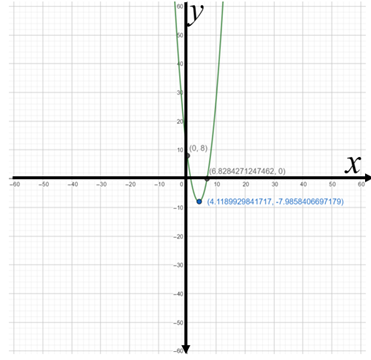# To Express: The quadratic function in standard form.### Precalculus: Mathematics for Calcu...

6th Edition
Stewart + 5 others
Publisher: Cengage Learning
ISBN: 9780840068071### Precalculus: Mathematics for Calcu...

6th Edition
Stewart + 5 others
Publisher: Cengage Learning
ISBN: 9780840068071

#### Solutions

Chapter 3.1, Problem 24E

a.

To determine

## To Express: The quadratic function in standard form.

Expert Solution

the quadratic function is expressed in standard form as  f(x)=1(x+1)22

### Explanation of Solution

Given: The function is f(x)=(x28x+8)

Calculation:

The quadratic function f(x)=(x28x+8) is expressed in standard form as:

f(x)=a(xh)2+k , by completing the square. The graph of the function f is a parabola with vertex (h,k)

The parabola opens upwards if a>0 .

Solve the function:

f(x)=(x28x+8) f(x)=1(x28x)+8                        [factor 1 the x terms] f(x)=1(x28x+16)+8(116)   [complete the square: add 16 to parentheses, subtract (116) outside] f(x)=1(x4)28                           [factor and multiply]

On comparing the above equation with standard form f(x)=a(xh)2+k ,

Therefore, the quadratic function is expressed in standard form as  f(x)=1(x4)28

b.

To determine

Expert Solution

### Explanation of Solution

Given: The function is f(x)=(x28x+8)

Graph:

The standard form of the function is:

f(x)=1(x4)28

From the standard form it is observed that the graph is a parabola that opens upward and has vertex (4,8) . As an aid to sketching the graph, find the intercepts.

The y-intercept=f(0)=8 and The x-intercept is 7 and 0 . The graph f is sketched in the figure below.

Use graphing calculator to graph the function: f(x)=(x28x+8)c.

To determine

### To Find: The maximum or minimum value of the function.

Expert Solution

The value of minima is f(4)=(8)

### Explanation of Solution

Given: The function is f(x)=(x28x+8)

Calculation:

From the above graph it is seen that the parabola opens upward, since the coefficient of x2 is positive, f has minimum value. The value of minima is f(4)=(8)

### Have a homework question?

Subscribe to bartleby learn! Ask subject matter experts 30 homework questions each month. Plus, you’ll have access to millions of step-by-step textbook answers!